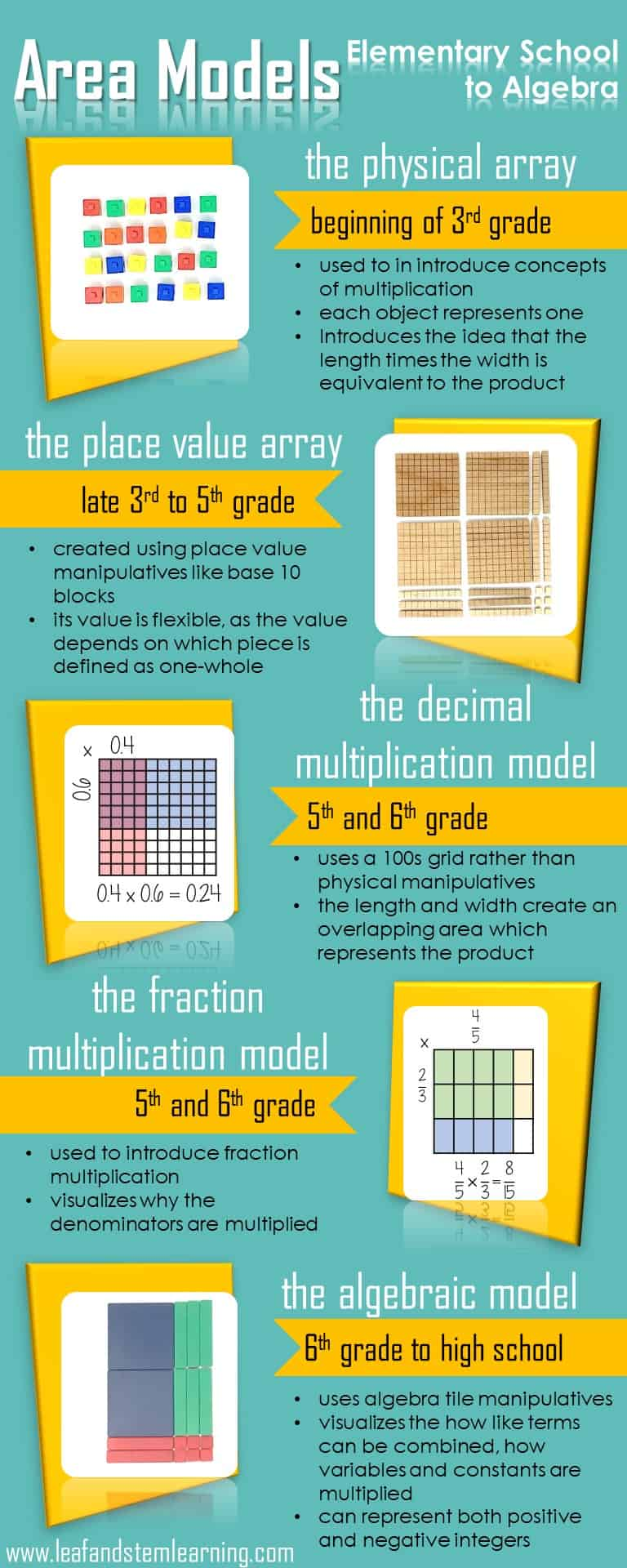When children are beginning to learn how to multiply numbers, one of the first things they learn is to make a pattern with objects in an array.  They count the manipulatives and notice that there is a length and a width.  That they can also count all of manipulatives to find a total.  From this early experience, students begin a first step toward a skill that will continue to build all the way through high school algebra.

When Common Core and other curriculums began emphasizing non-standard algorithms over the traditional methods that many adults used exclusively in school, there was backlash.  Memes and internet threads devoted to slamming these non-standard methods as overly cumbersome or inefficient popped up everywhere.  These missed the purpose of the teaching and learning of these methods, such as the area model in the mathematical development of our students.  Methods like area models are developed with the purpose of gaining a lasting understanding of the mechanics of math rather than simply the answer to a quick math problem.  The standard algorithm is often the most efficient way to solve a problem, but it often hides the reasoning of the math from students learning to do more complicated work at younger and younger ages.  Yes, the area model looks very different from the math many of us did as children, but the mechanics is the same.

Area models and arrays rely on one simple thought: the the length or a rectangle times its width will equal to the total area.  The first area model model that students use is a simple physical array.

This basic model is actually the foundation for learning that will continue through high school!  How can this model be used to further young students’ understanding?  The most important use of this model is the visual difference between what adding looks like compared to multiplication.  It makes it more clear how different 6 + 4 is from 6 x 4.  This distinction will be very important when students begin to study the order of operations.  Once students have mastered multiplication facts, they move on to two-digit multiplication.  This is where the models take the turn that many adults begin to be uncomfortable with the math!

Are your students having trouble taking the physical model into the algorithm?  Try this tip: Have your students build physical arrays on top of the multiplication chart.  This will help them see the relationship between the model that they are building and the facts they are working on learning!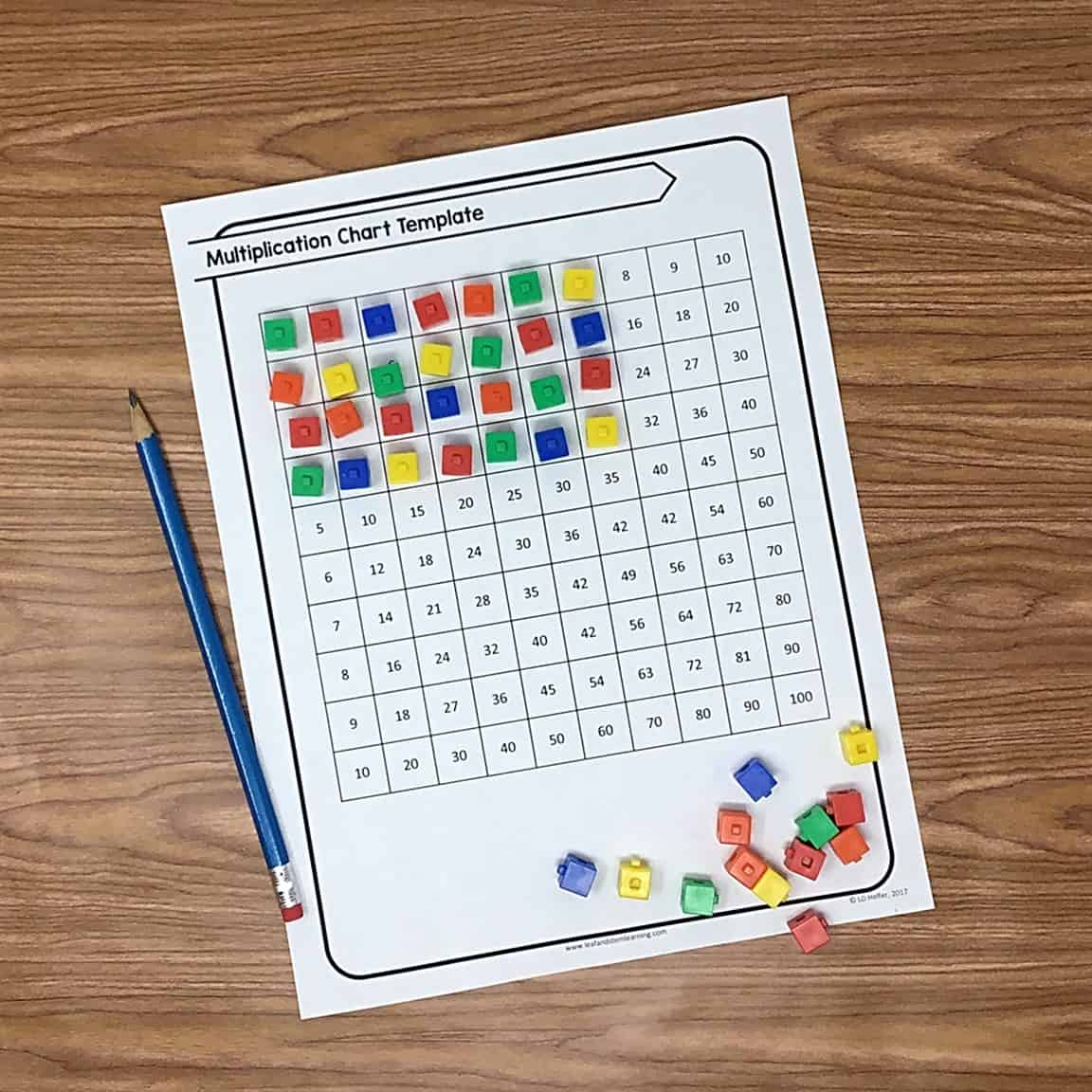Using manipulatives like base ten blocks to show place value relationships is the next step in the evolution of the area model.  This can method can be tricky for educators and parents who are not used to how the length and width relationships work within each of the units of the base ten blocks.  Another aspect of this model that can be difficult, is the ability of the blocks to represent different values.  When working with whole number multiplication, the unit cube represents one, but when working with decimals, the unit cube represents one-hundredth. Using place value modeling shows students why a zero must be placed when multiplying 2-digits by 2-digits.  It can also give students who are less confident with multiplication a bridge to get from 1-digit multiplication to more complex problems.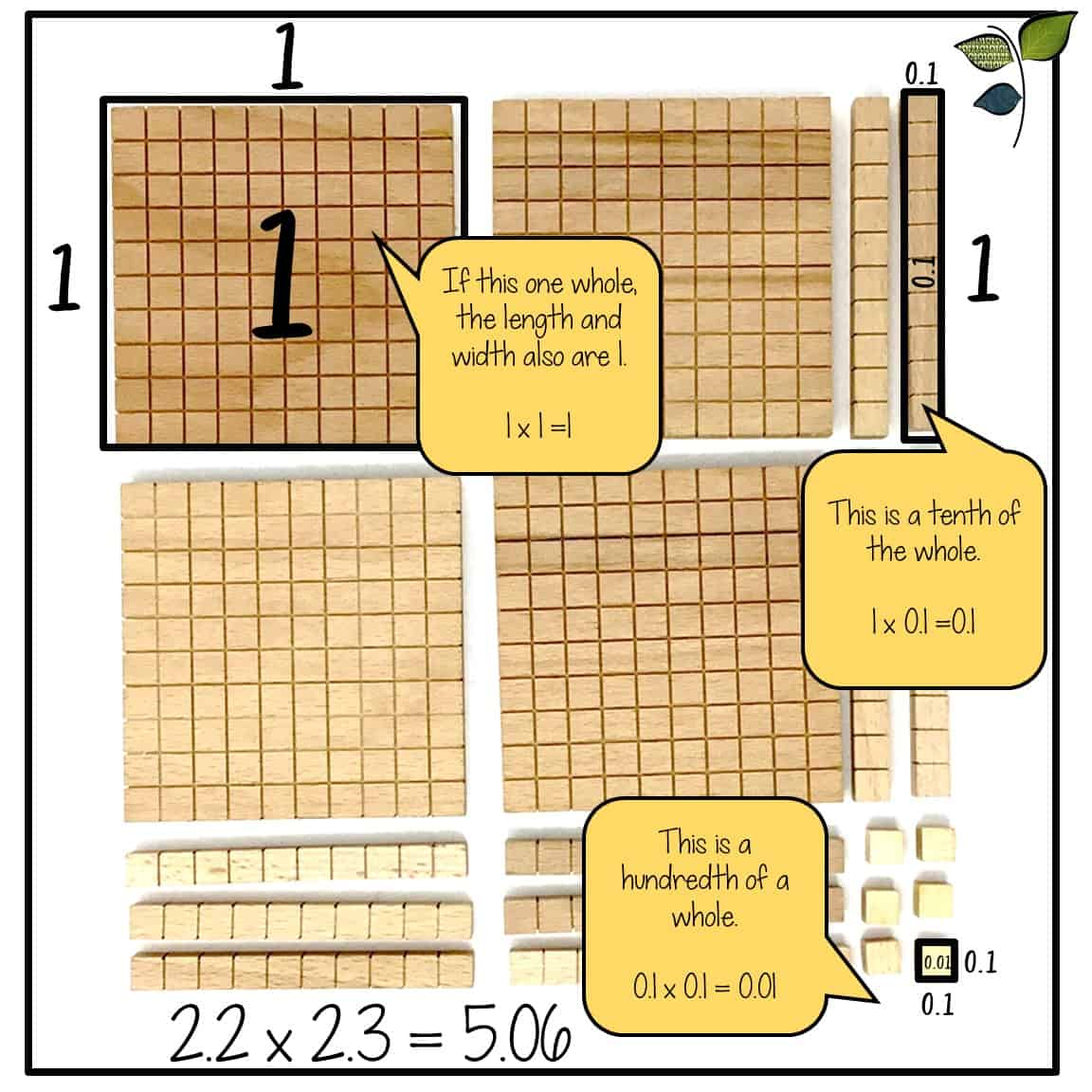Once students reach around fifth or sixth grade, the use of area models takes another transformation.  The concrete model moves toward a visual representation.  With decimals, this often takes the form of a hundreds grid.  Using this model is one of the best ways to give students understanding of why decimals are not lined up in a multiplication problem.  When students are exposed only to algorithm work, they often have trouble remembering when to line up decimals versus when to move a decimal.  Give them the understanding of the why of the decimal placement will help them have a more natural memory and understanding of the concept, and they will not need to rely on memorization as heavily.  Also, when students use an area model to represent fraction multiplication, they are able to visualize the reason for the multiplication of the denominators.  After learning how to add fractions, this is important because students have firmly implanted the idea of finding common denominators in their minds as necessary for working with fractions.  When multiplying, this of course, is not needed and will result in the wrong answer.  Again, like when working with decimals, many students get confused about the rule differences between additive and multiplicative operations.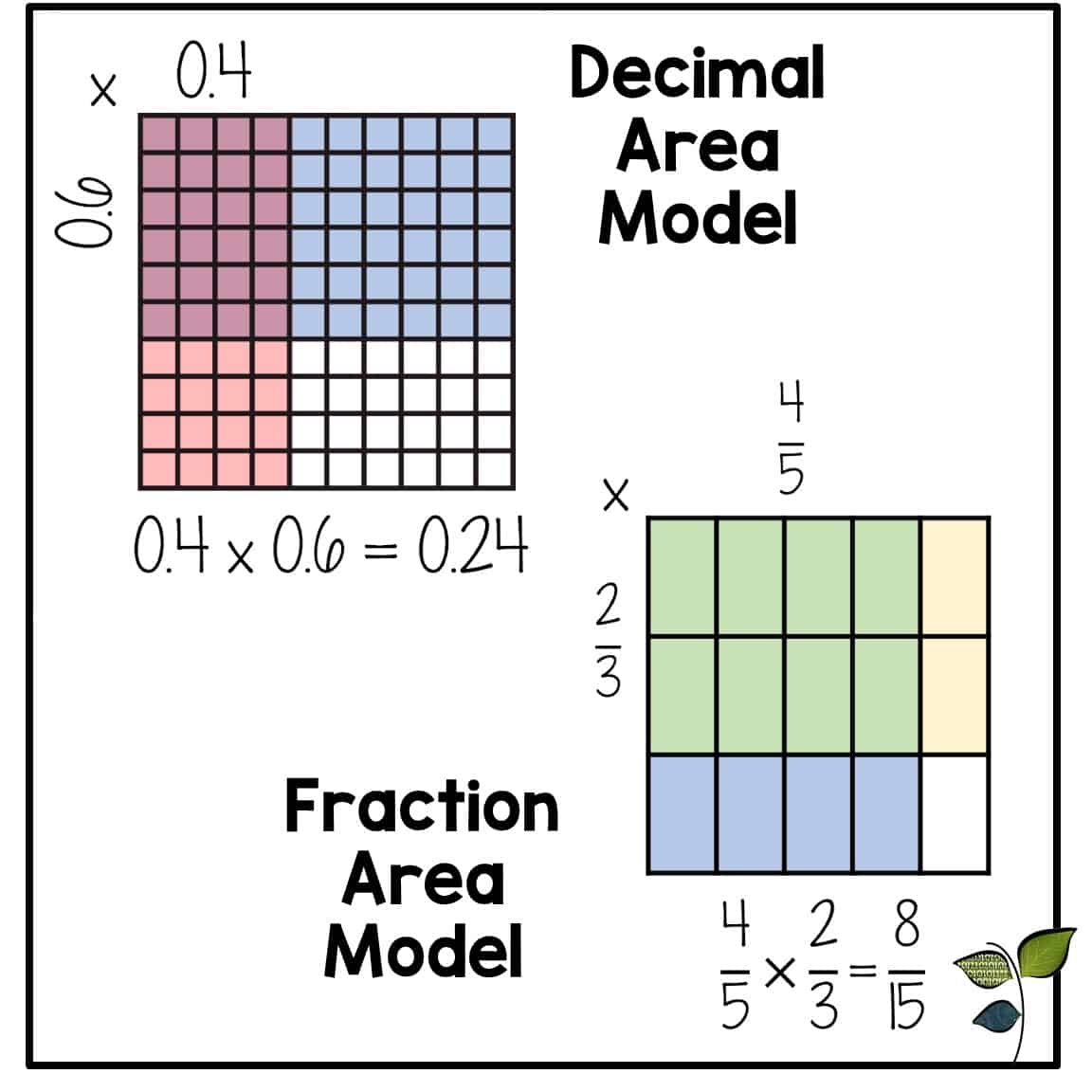Do your students have trouble seeing the length and width in decimal and fraction area models?  Try this tip: Draw number lines along the length and the width.  Mark the wholes first.  Then highlight the wholes to make unit squares so that the denominator can be easily counted.  Check out the video below to see the steps with a complex mixed number multiplication!

All of the previous models, though different, deal with numeric length and width.  Area models do not have to use numeric values and can be used to simplify algebraic expressions.  A manipulative called algebra tiles are commonly used to build algebraic area models.  Using an area model to simplify algebraic expressions can be used as an alternative to FOIL.  Although, many of us who are teaching now grew up using the FOIL method, a mnemonic that stands for first, outside, inside, last, to multiply algebraic expressions, this method has some obvious shortfalls.  One of the greatest is when one of the parentheses includes three terms instead of two.  The FOIL method only works if both multipliers have only two terms, but there is nothing that limits algebraic problems to two terms.  Students who have no other method other than FOIL will likely get stuck on a problem with no other method to use.Area models are an essential tool for fulling understanding multiplicative relationships.  From the first use to build multiplication facts all the way through algebra this model, although not what most of grew up with when learning math ourselves, are one of the best methods for creating a constant and understandable model of understanding for students.  Although the math grows more complex, each time it can be made to feel like it is something already known by using a familiar model of solving.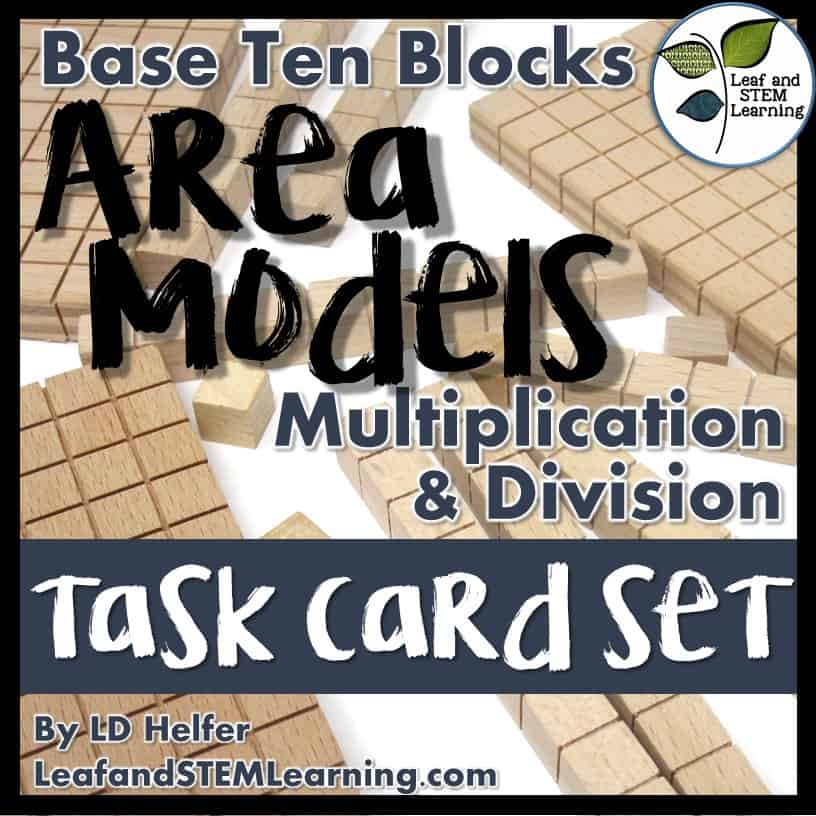Do you need materials to teach your students about whole number or decimal area models? Check out this task card set with bonus interactive notebook materials!